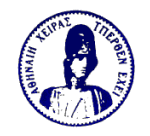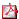SEMINARIOMATEMATICO E FISICODI MILANO

 150 years of Riemann Hypothesis RISM (Riemann International School of Mathematics) organizes the meeting ADVANCES IN NUMBER THEORY AND GEOMETRY Verbania (Italy), April 19-24, 2009

 Massimo Bertolini: On the Birch and Swinnerton-Dyer conjecture, I & II Abstract: We will begin by formulating the Birch and Swinnerton-Dyer conjecture, which relates the analytic properties of the L-series of an elliptic curve to certain arithmetic invariants of the curve, notably the rank of its group of rational points. We will then proceed to illustrate the known results on the Birch and Swinnerton-Dyer conjecture, and conclude by touching upon analogues and generalisations of this conjecture. Enrico Bombieri: The Classical Theory of Zeta and L-functions Abstract: Lecture I. Riemann's formula, zeta functions and L-functions. Summary: Beginning with Riemann's "Explicit Formula", the zeta function, Dirichlet L-functions, and the Dedekind zeta functions are introduced and their main properties are discussed. If time allows, zeta functions of modular forms and elliptic curves will be briefly introduced. Lecture II. The analytic theory of the zeta function, known and conjectural results. Families and random matrix theory. Summary: This lecture gives a survey of results about the analytic behavior of the zeta function. The conjectural correlations of zeros and the recent conjectures about moments derived from random matrix theory will also be mentioned briefly. Lecture III. The zeta function of curves over a finite field. Summary: This lecture is dedicated to the zeta functions of curves over a finite field and their analogy with the Dedekind zeta function. A brief sketch of Weil's first proof of the analog of the Riemann Hypothesis for them will be given. If time allows, Weil's formulation of the "Explicit Formula" will also be given. Mauro Carfora: Quantum fluctuations and Riemannian Geometry: The case of the Ricci flow Abstract: The talk will be focalized on the interplay among Ricci flow and quantum theory. After briefly introducing Ricci flow we discuss its connection with renormalization group analysis in quantum field theory, (I will make an effort to present this in a math. "reasonable" way). We will emphasize the suggestive applications that such a relation has both in Riemannian Geometry, and in improving our geometrical understanding of Quantum Field Theories. This is an active field of investigation providing a nice case of cross-fertilization between riemannian geometry and physics. Jeff Cheeger: Curvature bounds and applications to 4-dimensional Einstein metrics Abstract:(39 KB) Jean-Pierre Demailly: Singularities of currents and applications to algebraic geometry Lecture 1. Fundamental approximation theorem for plurisubharmonic functions Abstract: We will introduce basic invariants for plurisubharmonic singularities (Lelong numbers, singularity exponents) and will discuss a fundamental approximation result relying on the Ohsawa-Takegoshi extension theorem. Lecture 2. Analytic Zariski decomposition and related results in algebraic geometry Abstract: The goal of this second talk will be to outline an extension of Zariski decomposition, as known for surfaces, to higher dimensions, where it holds only approximately. This can be viewed as part of a new intersection theory framework which has deep applications to algebraic geometry - e.g. a characterization of the pseudoeffective cone and a characterization of projective algebraic varieties possessing a pseudoeffective canonical class. Lecture 3. Semicontinuity of singularities, estimates for Monge-Ampére operators and existence of Kähler-Einstein metrics Abstract: This third part will discuss a fundamental semicontinuity theorem for psh singularities, which can be used to extend Nadel's criterion for the existence of Kähler-Einstein metrics on Fano orbifolds. New results concerning log canonical thresholds in relation with estimates for Monge-Ampére operators will also be outlined, in connection with applications to local algebra and birational geometry. E. Kowalski: Some aspects and applications of the Riemann Hypothesis over finite fields Abstract: Since Weil's proof of the Riemann Hypothesis for curves over finite fields, and even more since Deligne's proof of his remarkable generalizations of the general Weil conjectures, these results have had numerous applications. We will present some of them, focusing particularly on those in analytic number theory, and we will highlight some of the important open questions still remaining. Raghavan Narasimhan: Bernhard Riemann: Remarks on his life and some of his mathematical work Abstract: The mathematical work I will discuss will be mainly that on the zeta function and his unpublished lectures on the hypergeometric series. Alberto Perelli: Non-linear twists of L-functions Abstract:(52 KB) Mario Rasetti: Topological invariants of 3-manifolds and quantum computation Abstract: After briefly reviewing the basic aspects of quantum information processing, based on topological quantum field theory methods, a quantum algorithm for approximating efficiently 3--manifold topological invariants in the framework of the SU(2) Chern--Simons--Witten topological quantum field theory at finite values of the coupling constant k will be discussed. The model of computation adopted is the q--deformed spin network model viewed as a quantum recognizer automaton, where each basic unitary transition function can be efficiently processed by a standard quantum circuit. The algorithm is an extension of that constructed for the (additive approximation) evaluation of polynomial invariants (Jones) of colored oriented links. All the significant quantities --partition functions as well as observables-- of the quantum field theory can be processed efficiently on a quantum computer, reflecting the intrinsic, field--theoretic solvability of such theory at finite k. The talks aims as well to providing a critical overview of some basic conceptual tools underlying the construction of the quantum invariants of knots, links and 3--manifolds and their connections with algorithmic questions that arise in geometry and quantum gravity models. Harold Rosenberg: Entire minimal graphs in H x R and the construction of surjective harmonic diffeomorphisms from the complex plane C to the hyperbolic plane H. Abstract: In the 1950's, Heinz proved there is no harmonic diffeomorphism from H onto C. He then used this to prove Bernsteins' theorem: An entire minimal graph over the euclidean plane is linear. It was conjectured by R. Schoen that there are no surjective harmonic diffeomorphisms from C onto H. We will construct such diffeomorphisms by constructing entire minimal graphs in H x R that are conformally C. This is joint work with Pascal Collin. Peter Sarnak: L-functions of modular forms and some applications Lecture 1: GL(2) Abstract: We review the classical theory of Hecke and Maass which generalizes Riemann's work on the zeta function to L-functions associated with modular forms in the upper half plane. In particular we pay attention to the converse theorem of Weil, its philosophical implications and higher symmetric power L-functions. Lecture 2: GL(n) Abstract: We give a quick introduction the theory of modular forms on GL(n). Via Riemann and Hecke's methods they produce "standard L-functions" which are expected to contain all such functions that arise in "nature". We discuss the basic conjectures associated with these forms and their L-functions that is the Grand Riemann Hypothesis and the Generalized Ramanujan Conjectures and we report on what is known towards them. Lecture 3: Subconvexity and some applications Abstract: After explaining the subcovexity problem for general L-functions and some methods that have been used to resolve it (mainly in GL(2)), we give applications via period formulae to local to global problems in the theory of integral quadratic forms and to the Quantum Unique Ergodicity Conjecture. Nina Snaith: A short history of Riemann and random matrices. Abstract: It has been almost 40 years since Montgomery and Dyson uncovered the similarity between statistics of the Riemann zeros and the eigenvalues of random matrices. In the past dozen or so years we have seen random matrix theory have a significant impact in number theory as we start to understand how to use it to attack some of the biggest questions concerning the Riemann zeta function and other L-functions. This talk will chart the rise of random matrix theory as a reputable means of studying Riemann's famous zeta function. Gudlaugur Thorbergsson: Singular Riemannian foliations and isoparametric submanifolds Abstract: In the talk I will explain both old and new results due to myself and others on singular Riemannian foliations that generalize or are analogous to isoparametric foliations in spheres and Euclidean spaces. I will also discuss the question when such foliations are homogeneous, i.e., when they are orbit foliations of isometric actions. Claire Voisin: Algebraic geometry versus Kaehler geometry. Abstract: Hodge theory is a common tool to study the topology of compact Kaehler or complex projective manifolds. We will explain in the first lecture how it can be used however to exhibit topological obstructions for a compact Kaehler manifold to admit a projective complex structure. Another very important difference between compact Kaehler manifolds and complex projective varieties comes from the absence of "motive" in the first case, possibly explaining the failure of the Hodge conjecture in the Kaehler setting. Here the motive is understood in the simplest sense, and provides a way of computing the cohomology of a projective variety by purely algebraic means. This will be the object of the second lecture.